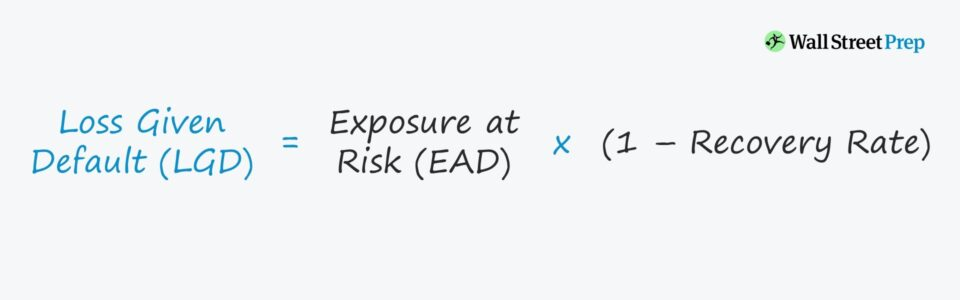Welcome to Wall Street Prep! Use code at checkout for 15% off.# Loss Given Default (LGD)

Guide to Understanding Loss Given Default (LGD)## How to Calculate Loss Given Default (Step-by-Step)

LGD, which stands for “loss given default”, measures the loss potential in the event of default, taking into consideration the borrower’s asset base and existing liens – i.e. collateral pledged as part of the lending agreement.

The loss given default (LGD) is the percentage of total exposure that is not expected to be recovered in the event of a default.

In other words, the LGD calculates the approximate loss on an outstanding loan, expressed as a percentage of the exposure at default (EAD).

In such a situation, the borrower is incapable of meeting interest expense or principal amortization payment requirements, which places the company in technical default.

Anytime that a lender agrees to provide financing to a company, there is a possibility that the borrower will default on the financial obligation, especially during economic downturns.

However, quantifying the potential losses is not as straightforward as assuming it is equal to the total value of the loan – i.e. the exposure at default (EAD) – because of variables such as collateral value and recovery rates.

For lenders projecting out their expected losses and how much capital is at risk, the LGD of their portfolio must be constantly monitored, especially if their borrowers are at risk of default.

## Liquidation Analysis: Collateral and Recovery Rates

The value of a borrower’s collateral and the recovery rates of the assets are critical factors that lenders such as financial institutions and banks must pay attention to.

• Collateral: Items with monetary value after liquidation (i.e. sold in the market for cash proceeds) that borrowers can pledge as part of a lending agreement to obtain a loan or a line of credit (LOC)
• Recovery Rates: An approximated range of recoveries that an asset would sell for in the market if sold now, depicted as a percentage of the book value

While the total capital provided as part of the loan agreement must be taken into account, the existing liens and contractual provisions are factors that can impact the expected loss.

As for the recovery rates of a company’s assets, the impact on a lender’s LGD is largely tied to where the debt tranche is in the capital structure (i.e. the priority of their claim – senior or subordinated).

In the event of a liquidation, the higher-ranking debt holders are more likely to receive full recovery because they must be paid first (and vice versa).

Putting the above together, the following rules tend to be true for lenders and their LGD:

• Liens on Borrower’s Collateral ➝ Reduced Potential Losses
• Higher Priority Claim in Capital Structure ➝ Reduced Potential Losses
• Large Asset Base with High Liquidity ➝ Reduced Potential Losses

## Loss Given Default Formula (LGD)

The loss given default (LGD) can be calculated using the following three steps:

• Step 1: In the first step to calculating the LGD, you must estimate the recovery rate of the claim(s) belonging to the lender.
• Step 2: Then, the subsequent step is to determine the exposure at default (EAD), which is the total capital contribution amount.
• Step 3: The final step in calculating the LGD is to multiply the EAD by one minus the recovery rate, as shown in the formula below.
Loss Given Default (LGD) = Exposure at Default * (1Recovery Rate)

Note that there are far more complex quantitative credit risk models out there to estimate the LGD (and related metrics), but we’ll focus on the simpler approach.

## Loss Given Default Calculation Example (LGD)

Let’s say, for instance, that a bank has lent \$2 million to a corporate borrower in the form of secured senior debt.

Due to underperformance, the borrower is currently at risk of defaulting on its debt obligations, so the bank is attempting to estimate how much it could lose in preparation for the worst-case scenario.

If we assume the recovery rate to the bank lender is 90% – which is on the higher end as the loan is secured (i.e. senior in the capital structure and backed by collateral) – we can calculate the LGD using the following formula:

• Loss Given Default (LGD) = \$2 million * (1 – 90%) = \$200,000

Therefore, if the borrower defaults, the estimated maximum loss incurred by the bank is around \$200k.

## Loss Given Default vs. Liquidity Ratios

Compared to liquidity ratios, such as the current ratio and quick ratio, LGD is different in that it does NOT depict how likely a borrower is going to default on an obligation.

LGD focuses instead on quantifying the potential negative impact to lenders in the event of default.

Note that LGD as a standalone metric fails to capture the probability that the default will actually occur.

• High LGDs signifies that the lender could lose a large sum of capital if the borrower were to default and file for bankruptcy.
• On the other hand, low LGDs are not necessarily positive, as the borrower could still be at a high risk of defaulting.

In closing, the key takeaway is that the loss given default (LGD) must be measured alongside other credit metrics to truly understand the actual risks attributable to a particular lender.Step-by-Step Online Course

### Everything You Need To Master Financial Modeling

Enroll in The Premium Package: Learn Financial Statement Modeling, DCF, M&A, LBO and Comps. The same training program used at top investment banks.